# Net Lorentz force, of a non-linear wire.

• PhiowPhi
In summary, the diagram shows two types of wires, a straight wire and a non-straight wire (zig-zag shaped wire), both placed in a magnetic field and with current flowing through them. The straight wire experiences a force towards the left, while the zig-zag wire experiences a force at an angle perpendicular to the current flow, depending on the angle of the wire. The force on the zig-zag wire can be calculated using the formula F = IL x B, but it will be less than the force on the straight wire due to the zig-zag wire's shorter length. The wires are assumed to have the same length and current flow, but it should be noted that if the wires are connected to a constant voltage source,

#### PhiowPhi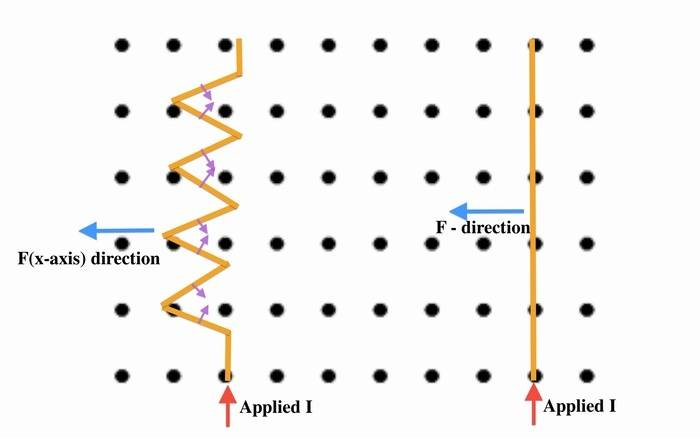From the diagram above, there are two types of wires a straight wire and a non-straight wire(zig-zag shaped wire), that are both placed inside a magnetic field(B), and have current flowing in both of them as well. The straight wire will experience a force and will move towards the left as marked(F-direction), while as the zig-zag wire will have the force dependent on the angle and Lorentz force, since there are a couple of bends on the zig-zag wire, there will be a force at an angle perpendicular to the current flow,as marked(purple arrows), the y-axis of each bend cancels out with the upper wire only leaving the x-axis remaining. Correct?
Depending on the angle(in degrees) the force on that zig-zag wire would be:

$$F = IL \times B$$ - which is the same as the straight wire, however , $$F_z = F_L \times \cos(x°)$$

Where $$F_L$$ is the Lorentz force of the zig-zag wire, and $$F_z$$ is the net-force of the zig-zag shaped wire's x-axis if the angle is known.

Is this correct? I don't think the second wire is equal to the straight wire, because there are some opposing forces that cancel each other out(y-axis) due to the current flow being opposite, and leaving some force remnant that is the x-axis.

**NOTE:** Both wires are the same in Length, and have the same current flow, and placed in the same magnetic field.

That is an interesting problem. I don't know the answer immediately. What I would do is to simplify the problem. Calculate the force on one zig zag, and see if that differs from the straight wire. I suspect that it will.

DaleSpam said:
That is an interesting problem. I don't know the answer immediately. What I would do is to simplify the problem. Calculate the force on one zig zag, and see if that differs from the straight wire. I suspect that it will.

It really is, I've been trying to figure this out for a bit. I initially assumed that it would be non-equal to the straight-wire. In fact, it should be less.
I broke it down one by one to calculate each individual zig-zag's force. But what I'm not sure of is the length equal to the wire like so: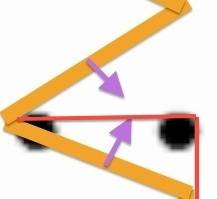Just the right side vertical red line.
OR: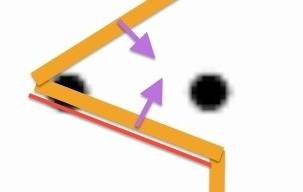That red line, blow the wire being the length for the Lorentz force, I assume it's the first one above.
The angle of force of that individual wire seems to be at an angle 60 degrees, or possibly less?
I should then add up the x-axis forces since the y-axis cancel out(because the current flow is in opposition).

@jim hardy , what's you're opinion on this?

PhiowPhi said:
But what I'm not sure of is the length equal to the wire like so:
I would not assume equal lengths of wire, I would allow the length of each to vary and see what effect it has on the force. It may be that the force cancels out for equal lengths but not for unequal lengths.

I would also do this for an arbitrary half-angle. However, there's something else to consider - are these wires connected to a constant voltage source or a constant current source? If the former, there will be less current flowing through the zig-zag.

Same net force.

Hmmmm
it wasn't clear to me whether the force on your wire is the force resulting from current flowing in the wire,
or is an externally applied force causing the wire to move.

Take a look here: It's one of those little confusing points:

http://hyperphysics.phy-astr.gsu.edu/hbase/magnetic/forwir2.html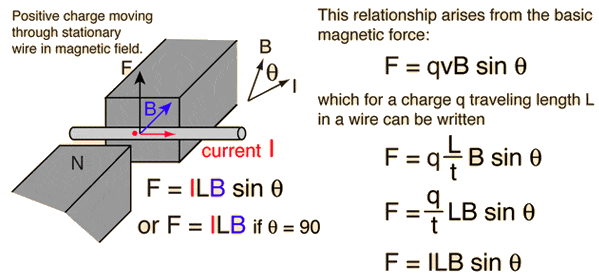Aha we're in luck - velocity and charge resolve to current !

So if you're figuring force resulting from current,
1. the vertical components of force cancel leaving only horizontal force.
2. the horizontal components of force add, .

Now your purple arrow has magnitude F = BLI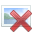where L is length of the zig , your red line
so we can resolve that force into a horizontal and a vertical component
let theta = angle between zig and horizontal
Fvertical = Fsin(theta)
and Fhorizontal = Fcos(theta)

Maybe this is the rub:
PhiowPhi said:
**NOTE:** Both wires are the same in Length, and have the same current flow, and placed in the same magnetic field.
Aha - if the wires are same length
then the zigzag structure must be not so tall as the straight one, unlike your sketch...
So, your zigzag spans less vertical run than the straight one;
hence cuts fewer lines of flux,
so experiences less force from the same current.

am i on right track?

I was initially confused by your arrows.. do they rigorously follow right hand rule and QVcrossB ?

old jim

•PhiowPhi
DaleSpam said:
I would not assume equal lengths of wire, I would allow the length of each to vary and see what effect it has on the force. It may be that the force cancels out for equal lengths but not for unequal lengths.

I'm not sure how to figure out or "measure" lengths properly for such a wire. It's true that the zig-zag wires are longer and have "more copper" if that makes sense.
But I was curious specifically for equal lengths, wanted to see the effect if they we're equal, why might equal lengths cancel while as non-equal lengths do not?

I would also do this for an arbitrary half-angle. However, there's something else to consider - are these wires connected to a constant voltage source or a constant current source? If the former, there will be less current flowing through the zig-zag.

Interesting, never thought about the type of power source, it's mostly likely a CCS. Why would they differ?
I assume there would be less current flowing in the zig-zag due to higher resistance since it's "longer" than the straight wire?

zoki85 said:
Same net force.

Could you explain why? I disagree.

jim hardy said:
Hmmmm
it wasn't clear to me whether the force on your wire is the force resulting from current flowing in the wire,
or is an externally applied force causing the wire to move.

Ops, sorry for not clearing that out, but it's from a current source(CCS to be exact).

jim hardy said:
Take a look here: It's one of those little confusing points:

http://hyperphysics.phy-astr.gsu.edu/hbase/magnetic/forwir2.htmlAha we're in luck - velocity and charge resolve to current !

So if you're figuring force resulting from current,
1. the vertical components of force cancel leaving only horizontal force.
2. the horizontal components of force add, .

Somewhat my analysis, but I was not sure If I was on the right track or not, seems that I am.

jim hardy said:where L is length of the zig , your red line

Aha - if the wires are same length
then the zigzag structure must be not so tall as the straight one, unlike your sketch...
So, your zigzag spans less vertical run than the straight one;

Well, I was not sure how to "measure" length properly. Therefore, sketched it to be the same as the vertical wire, but indeed it seems to be un-equal.
I must take the same length as the straight wire then form the zig-zag shapes.

jim hardy said:
am i on right track?

I was initially confused by your arrows.. do they rigorously follow right hand rule and QVcrossB ?

old jim

I believe so, I'm somewhat on the same track(aside from the confusion of lengths). And yes, the arrow are based on the right hand rule and QVcorssB.

PhiowPhi said:
why might equal lengths cancel while as non-equal lengths do not?
I don't know, I haven't worked the problem. The point is that if you leave the length of each as a variable when you do the math then you can substitute in equal or unequal numbers and see what happens. But if you assume equal values and do the math then you cannot know what happens for unequal lengths.

It isn't that I have a particular reason to assume either outcome. It is simply a problem solving strategy. Solve the most general problem that is tractable.

•PhiowPhi
PhiowPhi said:
Interesting, never thought about the type of power source, it's mostly likely a CCS. Why would they differ?
I assume there would be less current flowing in the zig-zag due to higher resistance since it's "longer" than the straight wire?

Exactly.

•PhiowPhi
DaleSpam said:
I don't know, I haven't worked the problem. The point is that if you leave the length of each as a variable when you do the math then you can substitute in equal or unequal numbers and see what happens. But if you assume equal values and do the math then you cannot know what happens for unequal lengths.

It isn't that I have a particular reason to assume either outcome. It is simply a problem solving strategy. Solve the most general problem that is tractable.

I agree! Well said.

Also, in relation to the Lorentz force I applied faraday's law of induction to this as well, if such a wire(zigzag) moved with velocity(v) in a magnetic field. When solving the issue of "length" that I had, it's quite easy to apply $$\epsilon = -vBL$$ to figure out induced EMF of that conductor.

It's really fun to solve "un-usual" problems, it helps solidify my understanding more and go beyond with the theory, therefore, appreciation of nature is amplified.

jim hardy said:
where L is length of the zig , your red line
so we can resolve that force into a horizontal and a vertical component
let theta = angle between zig and horizontal
Fvertical = Fsin(theta)
and Fhorizontal = Fcos(theta)

i think i might have swapped cos and sin, not sure - that's what i get for not drawing it out on paper...

but the approach will work

i do apologize for not spending the time to give a more thorough answer. Am time-sharing between PF and grandchildren,, today we were doing model rockets. Grand-daughter shows unusual mechanical aptitude for a seven year old...

PhiowPhi said:
It's really fun to solve "un-usual" problems, it helps solidify my understanding more and go beyond with the theory, therefore, appreciation of nature is amplified.
Bravo - keep up the good work. It certainly makes one more adept at solving problems by just applying his basics... and that is power!

old jim

I don't know, whether I understand the drawing in #1 right. Usually the dots indicate a vector pointing out of the plane, and if this is the magnetic field and the arrow labeled ("applied I") indicates the direction of the current density then the force should be to the right, not the left.

Now we need to clarify some very important basics: A current is a scalar and thus has no direction. Its sign is determined by the choice of the orientation of the crossectional surface it runs through and the current-density vector:
$$I=\int_{A} \mathrm{d}^2 \vec{f} \cdot \vec{j}.$$
The current density is a vector, given by ##\vec{j}=q \vec{v}##, where ##q## is the charge of the particles making up the electric current density, i.e., usually electrons in a usual metallic wire and ##\vec{v}## the flow-velocity field of these charges.

Now to the problem with the zig-zag wire. I think it's a good idea to look at the one zig-zag piece drawn in #7. So let's set up this problem a bit more carefully: We use a cartesian coordinate system with the ##x_1##-axis pointing to the right, the ##x_2##-axis pointing up in the paper plane and the ##x_3## axis pointing out of the paper plane, so that we have righthanded Cartesian basis, ##\vec{e}_1 \times \vec{e}_2=\vec{e}_3##.

Now the current density in the lower piece of the one zig-zag piece is
$$\vec{j}_1=\frac{I}{A} (-\cos \alpha \vec{e}_1+\sin \alpha \vec{e}_2)$$
and the upper one
$$\vec{j}_2=\frac{I}{A}(\cos \alpha \vec{e}_1+\sin \alpha \vec{e}_2).$$
Here ##I## is the current and ##A## the crossectional area of the wire; ##\alpha## is the angle of the zig-zag pieces with the ##x_1## axis.
The force density is
$$\vec{f}=\vec{j} \times \vec{B}=B \vec{j} \times \vec{e}_3.$$
For the first piece thus it's
$$\vec{f}_1=\frac{I B}{A} (\cos \alpha \vec{e}_2+\sin \alpha \vec{e}_1)$$
and the second
$$\vec{f}_2=\frac{I B}{A}(-\cos \alpha \vec{e}_2+\sin \alpha \vec{e}_1).$$
The total force is the volume integral of this with the volume given by the wire. Thus the net force of the two pieces is
$$\vec{F}=\ell A (\vec{f}_1+\vec{f}_2)=2\ell I \sin \alpha \vec{e}_1,$$
where ##\ell## is the length of each zig-zag piece. The net force thus is the same as for the straight wire. This case one gets for ##\alpha=\pi/2##. In this case, for the total force on the entire straight wire you have to set ##\ell=L/2##, and for an arbitrary ##\alpha## you have ##L=2 n \ell \sin \alpha##, where ##n## is the number of zig-zag's making up the left wire.

•jim hardy and PhiowPhi
PhiowPhi said:
Could you explain why? I disagree.
Explanation in vanhees71's post

vanhees71 said:
Usually the dots indicate a vector pointing out of the plane, and if this is the magnetic field and the arrow labeled ("applied I") indicates the direction of the current density then the force should be to the right, not the left.

His little purple force arrows are in agreement with the vector convention i was taught,
colloquially: a dot is the head of an arrow, as if coming out of the page;
a cross is the tailfeathers of an arrow going into the page.
His horizontal blue force vectors are as you said, reversed if using my convention.
I never thought about current being a scalar, or if i did I've forgotten...
Is that because it's a dot product?

I see it's been discussed before..

I suppose so long as the wire is small one gets away with treating current as if it were a vector, as in that Hyperphysics link?
http://hyperphysics.phy-astr.gsu.edu/hbase/magnetic/imgmag/forcurt.gif
We who grew up studying circuits should be aware of that "simplification".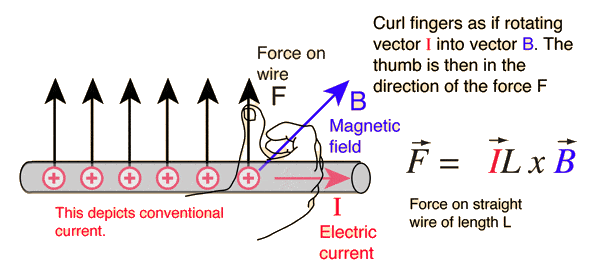Another refinement - thanks, vanhees.

Last edited:
This figure looks right, but please never ever say the current is a vector as indicated by the red vector in the magnetic-force formula. This is highly misleading.

The current density is a vector, which can be defined in a fluid picture (a good concept for classical electromagnetics) of charges flowing around. Then you have a charge density ##\rho(t,\vec{x})##, which gives the charge per unit volume around ##\vec{x}## at time ##t##. Then the current density is ##\vec{j}(t,\vec{x})=\rho(t,\vec{x}) \vec{v}(t,\vec{x})##, where ##\vec{v}(t,\vec{x})## is the flow-velocity field of the charges.

The current is defined as the charge flowing through a surface per unit time. If ##A## is this surface and ##\mathrm{d}^2 \vec{f}## the surface-area vectors, whose magnitude gives the size of the infinitesimal surface element at the given point and its direction is perpendicular to the surface pointing in one of two possible directions. Which you choose is arbitrary. Then the current is the scalar
$$I(t)=\int_{A} \mathrm{d}^2 \vec{f} \cdot \vec{j}(t,\vec{x}).$$
If you change the direction of the surface-element vectors, i.e., the orientation of the surface, ##I## flips sign, but it has no direction.

Now in a certain sense, your formula is still correct. It applies to the typical case of a thin wire. At each instant of time, you can consider the current distribution as homogeneous across the wire's cross-sectional area. Then denoting with ##\vec{t}## the tangent-unit-vector field along the wire, the current density is
$$\vec{j}(t,\vec{x})=\frac{I(t)}{A} \vec{t}(t,\vec{x}).$$
Here, the orientation of the cross-sectional areas along the wire is given by the choice of the direction of the unit-tangent vectors, which are perpendicular to the cross section. Then the equations of motion (or Poynting's theorem) tells you that the force density due to a magnetic field is
$$\vec{f}(t,\vec{x})=\vec{j} \times \vec{B}.$$
To get the total force on the wire, you have to take the volume integral of this. Since the current only flows along the wire and since you can choose the volume elements as ##\mathrm{d}^3 \vec{x}=A \mathrm{d} ## you get
$$\vec{F}(t)=\int_{0}^L \mathrm{d} l A \vec{j} \times \vec{B}=I(t) \int_0^{L} \mathrm{d} l \vec{t} \times \vec{B}.$$
The latter you can write as a line integral along with the curve given by the wire with the (arbitrarily chosen!) orientation given by ##\vec{t}##:
$$\vec{F}(t)=\int_{\text{wire}} \mathrm{d} \vec{x} \times \vec{B}.$$
Now to the figure in #1. With the standard Cartesian coordinates and with the standard meaning of the dots as pointing out of the paper we have (for the straight wire) ##\vec{B}=B \vec{e}_3## (##B>0##), ##\mathrm{d} \vec{x}=\mathrm{d} x \vec{e}_2## and thus, for a homogeneous magnetic field
$$\vec{F}=I L B \vec{e}_2 \times \vec{e}_3=I L B \vec{e}_1.$$
So the force is pointing in the positive ##x_1## direction, i.e., to the right and not to the left as drawn in this picture.

•PhiowPhi and jim hardy
vanhees that's elegant.
I remember working with current density and fields in early physics classes, and one on semiconductor physics.
Obviously i got quite rusty.
In circuit analysis the charges are constrained to the wires so one doesn't use his fields, just Ohm's law.
Then he hits machinery courses where the magnetic circuits do require thinking in fields... i think that's why magnetics is baffling for so many EE's.

Thanks.

old jim

@vanhees71

That is just beyond me, I'm currently studying what you've replied.
My apologize for the incorrect direction of force. I didn't mean to have current seem to be a vector, just wanted to point out how I used the right-hand rule to figure out the direction of force(which was incorrect).

Could you please "dumb-down" you're conclusion to a beginner like me? I'm looking at the Math of F-1 and F-2 then the (net-F) and cannot understand as to why the Lorentz force of the straight wire and the zig-zag one are equal?
From the Math, I find them un-equal and for the zig-zag wire due to the orientation of the conductors and the flow of current it might be less? My apologize for not following along the Mathematic's provided, but I'm not yet capable of understanding & appreciating them yet.

What precisely is it what you don't understand? Perhaps Should I prepare a drawing with the geometry of the one zig-zag piece? It's really simple geometry, and the vectors should help with the calculation concerning the directions.

vanhees71 said:
What precisely is it what you don't understand?

Well, as to how the non-linear wire(zig-zag) has the same Lorentz force as the linear one(Assuming all variables we're equal, but the orientation). What was intuitive to me is that they would differ?

vanhees71 said:
Perhaps Should I prepare a drawing with the geometry of the one zig-zag piece? It's really simple geometry, and the vectors should help with the calculation concerning the directions.

Please, it might help me understand more.

jim hardy said:
vanhees that's elegant.
I remember working with current density and fields in early physics classes, and one on semiconductor physics.
Obviously i got quite rusty.
In circuit analysis the charges are constrained to the wires so one doesn't use his fields, just Ohm's law.
Then he hits machinery courses where the magnetic circuits do require thinking in fields... i think that's why magnetics is baffling for so many EE's.

Thanks.

old jim
The most trouble I had with AC circuit theory was to get the signs right. Then I once taught 2nd semester engineering students about it and thought, how to make this trouble easier. Then I realized that one should use vectors consistently everywhere and then it's all about the right-hand rule in the integral theorems by Gauß and Stokes, and those are always with the right-hand rule of the one or the other kind. I keep the corresponding website. So you may have a look:

http://fias.uni-frankfurt.de/~hees/physics208.html

PhiowPhi said:
Well, as to how the non-linear wire(zig-zag) has the same Lorentz force as the linear one(Assuming all variables we're equal, but the orientation). What was intuitive to me is that they would differ?

Please, it might help me understand more.
Here it is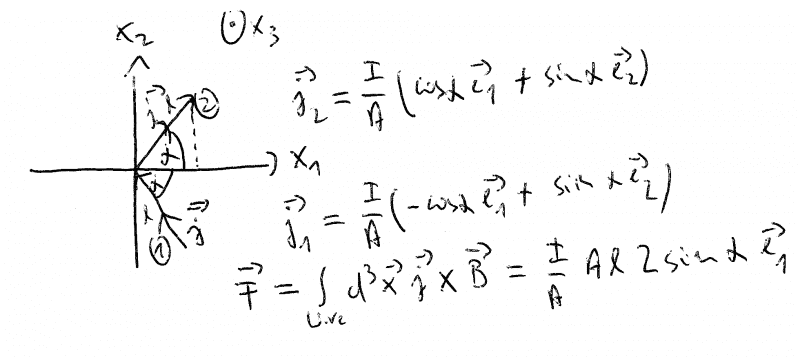Thank you @vanhees71 I'm going to study this, and compare it to the straight wire.
I edited the image for the force vectors is this correct: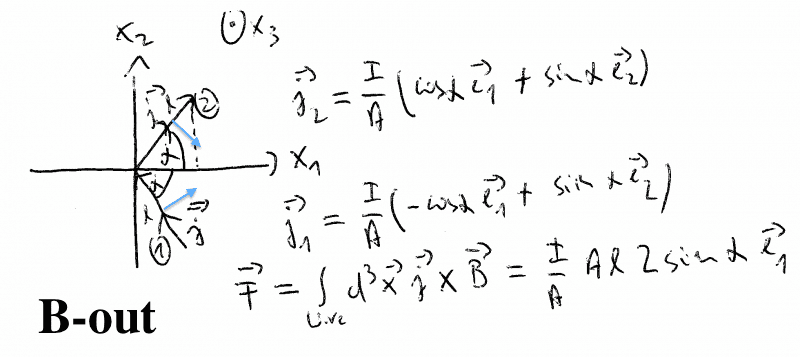Where those blue arrows are the Lorentz force per part(upper/lower).

Yes. You have for the force densities
$$\vec{f}_1=\vec{j}_1 \times \vec{B}=\frac{I B}{A} (-\cos \alpha \vec{e}_1+\sin \alpha \vec{e}_2) \times \vec{e}_3=\frac{IB}{A} (+\cos \alpha \vec{e}_2+\sin \alpha \vec{e}_1,$$
$$\vec{f}_2=\vec{j}_2 \times \vec{B}=\frac{I B}{A} (+\cos \alpha \vec{e}_1+\sin \alpha \vec{e}_2) \times \vec{e}_3=\frac{IB}{A} (-\cos \alpha \vec{e}_2+\sin \alpha \vec{e}_1.$$
I've just used the right-handedness and orthonormality of the Cartesian basis, ##\vec{e}_1 \times \vec{e}_3=-\vec{e}_2## and ##\vec{e}_2 \times \vec{e}_3=+\vec{e}_1##.

@vanhees71

Hmmm in post 13